# How to Calculate and Solve the Centre of Gravity of a Segment of a Sphere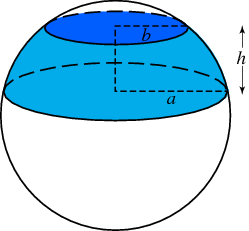The image above represents a segment of a sphere.
To compute the centre of gravity of a segment of a sphere requires two essential parameters. These parameters are the radius of the sphere and height of the segment of the sphere.

The formula for computing the centre of gravity of a sphere is:

C.G. = 3(2r – h)² / 4(3r – h)

Where:
C.G. = Centre of Gravity
r = Radius of the Sphere
h = Height of the Segment of the Sphere

Let’s solve an example
Find the centre of gravity of the segment of the sphere where the radius of the sphere is 10 m and the height of the segment of the sphere is 4 m.

This implies that:
r = Radius of the Sphere = 10
h = Height of the Segment of the Sphere = 4

C.G. = 3(2(10) – 4)² / 4(3(10) – 4)
C.G. = 3(20 – 4)² / 4(30 – 4)
C.G. = 3(16)² / 4(26)
C.G. = 3(256) / 104
C.G. = 768 / 104
C.G. = 7.38

Therefore, the centre of gravity of the segment of the sphere is 7.38.

Nickzom Calculator – The Calculator Encyclopedia is capable of calculating the centre of gravity of a segment of a sphere at a height, h at a distance from the centre of the sphere measured along the height.

To get the answer and workings of the center of gravity of a segment of a sphere using the Nickzom Calculator – The Calculator Encyclopedia. First, you need to obtain the app.

You can get this app via any of these means:

To get access to the professional version via web, you need to register and subscribe for NGN 1,500 per annum to have utter access to all functionalities.
You can also try the demo version via https://www.nickzom.org/calculator

Once, you have obtained the calculator encyclopedia app, proceed to the Calculator Map, then click on Mechanics under the Engineering section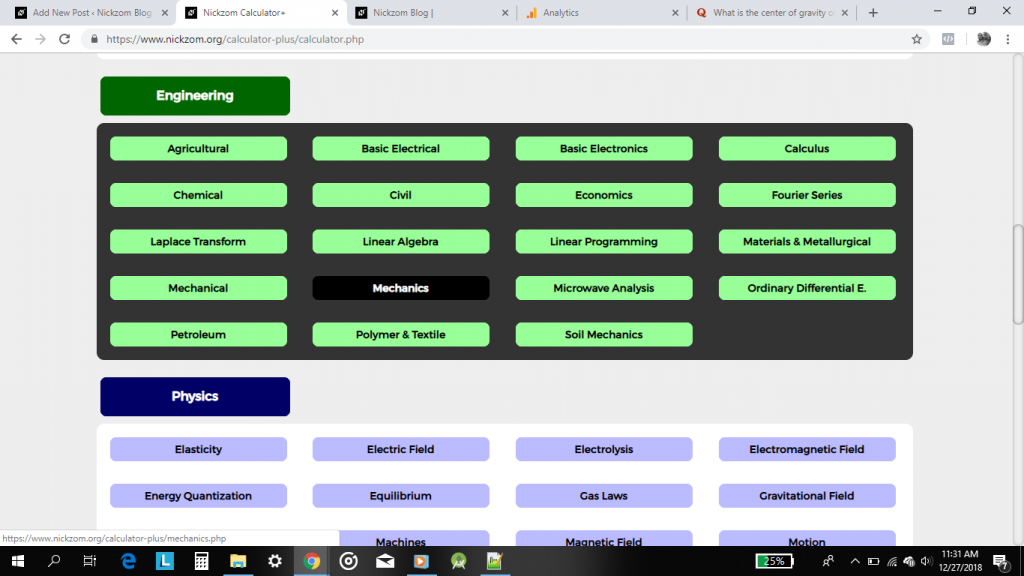Now, click on Centre of Gravity under Statics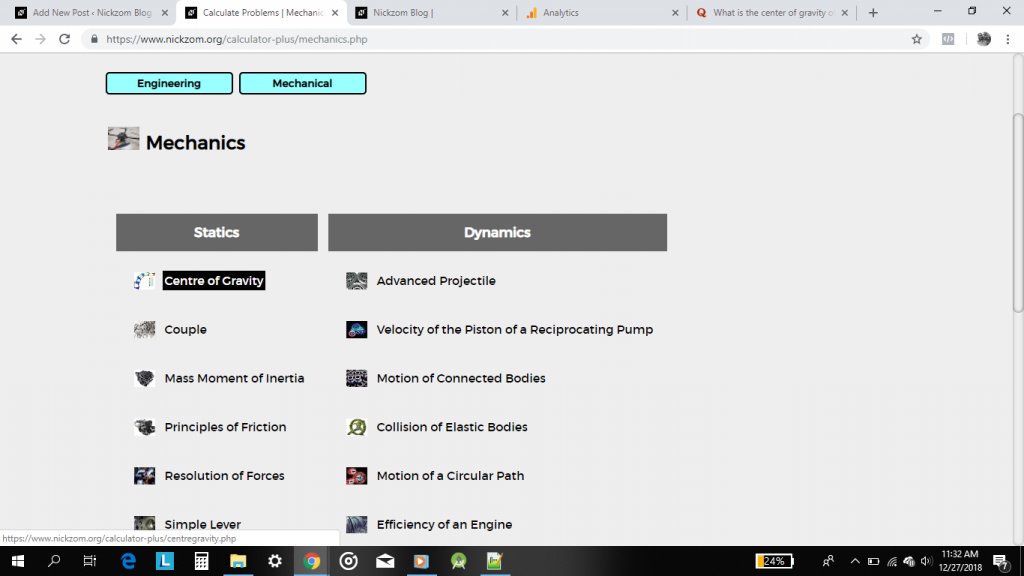Now, click on Centre of Gravity of a Segment of a Sphere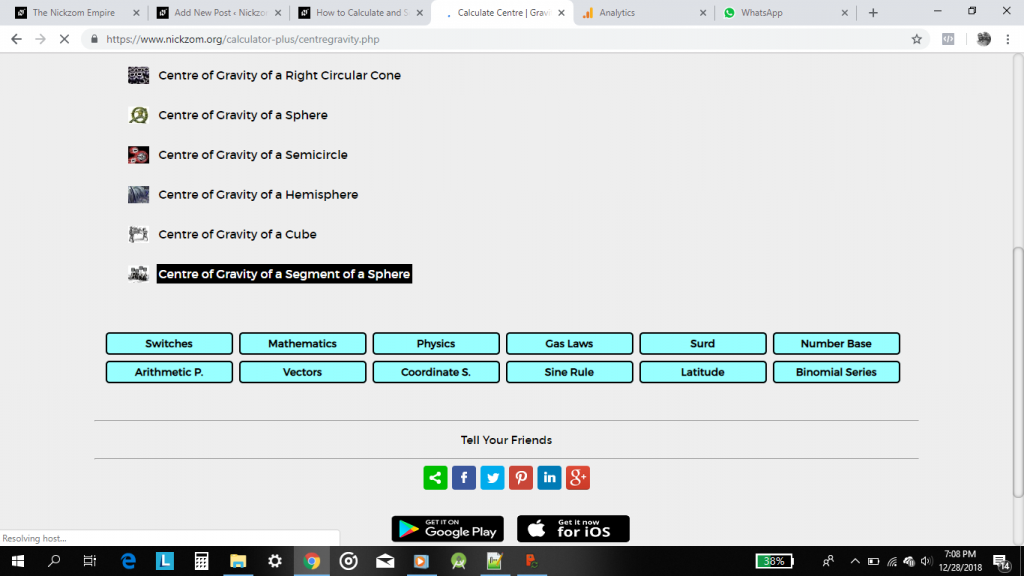The screenshot below displays the page or activity to enter your values, to get the answer for the centre of gravity of a segment of a sphere according to their respective parameters which are the radius of the sphere (r) and the height of the segment of the sphere (h).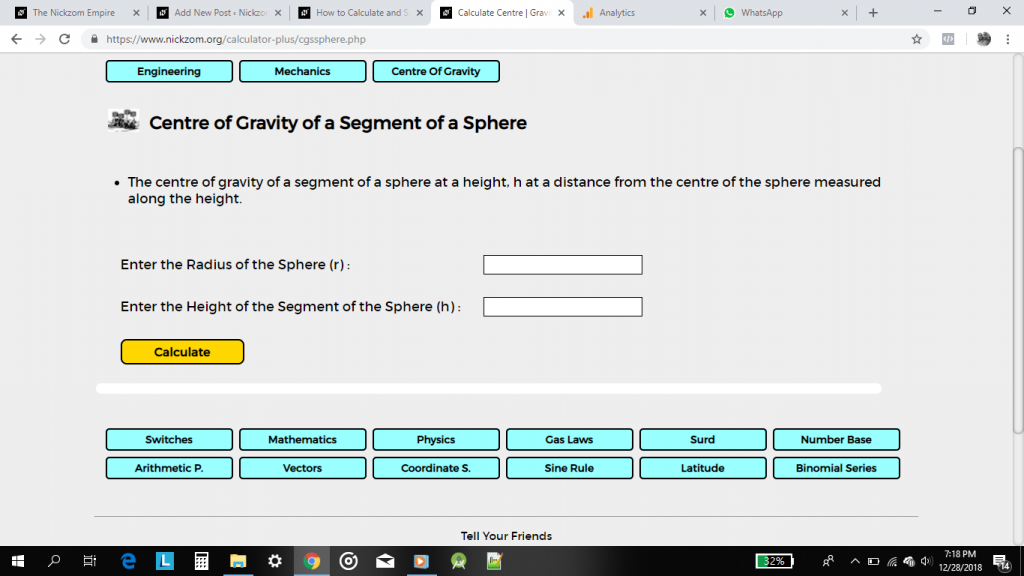Now, enter the values appropriately and accordingly for the parameters as required by the example above where the radius of the sphere is 10 and the height of the segment of the sphere is 4.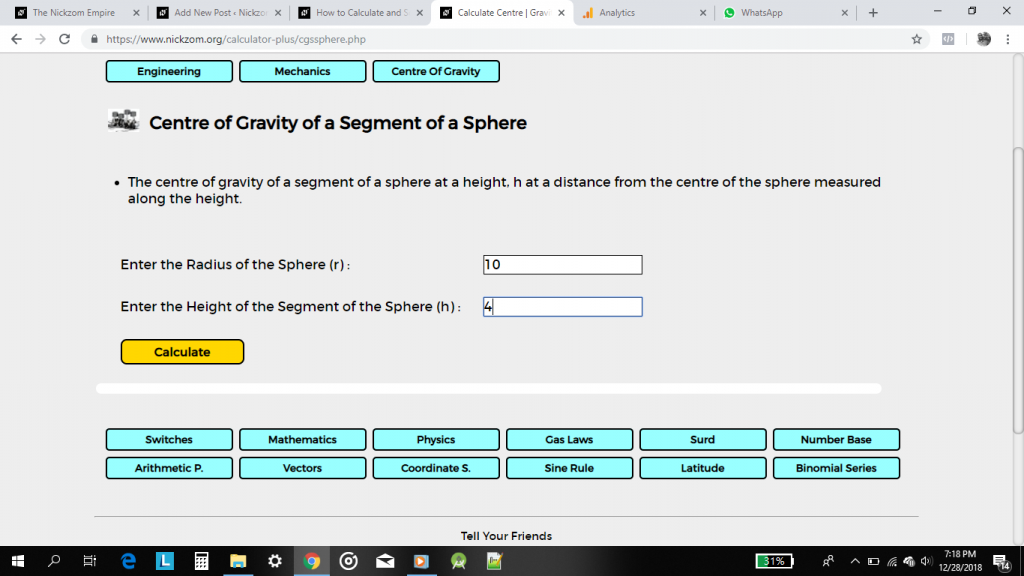Finally, click on the Calculate button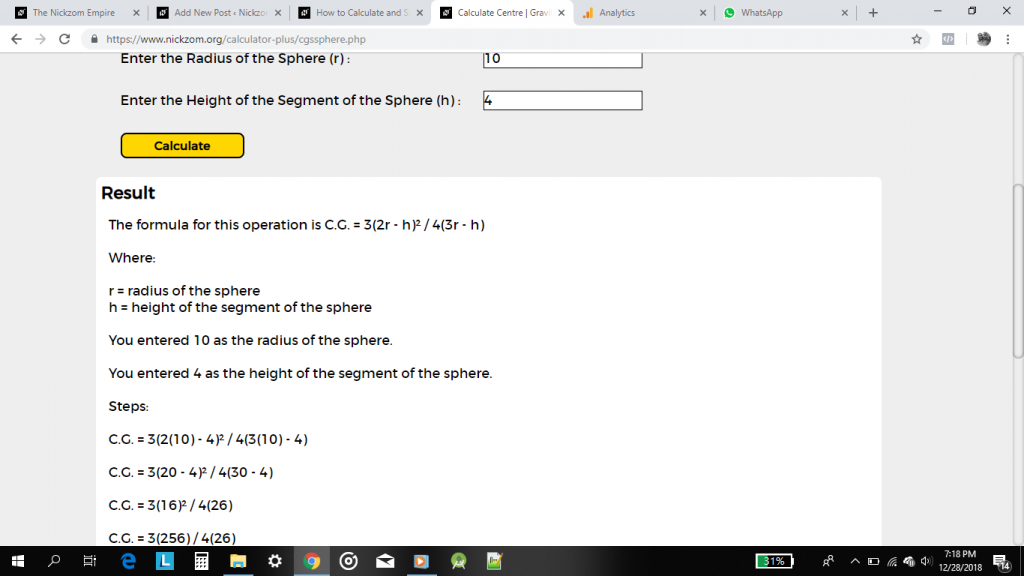As you can see from the screenshots above, Nickzom Calculator – The Calculator Encyclopedia solves for the centre of gravity of a segment of a sphere and presents the formula, workings and steps too.